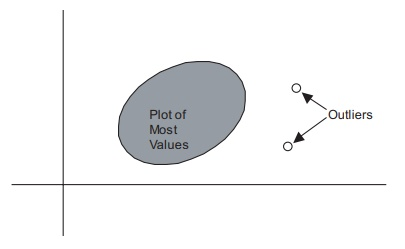# Insensitivity of Rank Tests to Outliers

| Home | | Advanced Mathematics |

## Chapter: Biostatistics for the Health Sciences: Nonparametric Methods

Outliers are unusually large or small observations that fall outside the range of most of the measurements for a specific variable.

INSENSITIVITY OF RANK TESTS TO OUTLIERS

Outliers are unusually large or small observations that fall outside the range of most of the measurements for a specific variable. (Outliers in a bivariate scatter plot were illustrated in Chapter 12, Figure 12.4) Outliers impact the parametric tests that we have studied in the previous chapters of this text; for example, Z tests and t tests for evaluating the differences between two means; ANOVAs for evaluating the differ-ences among three or more means; and tests for nonzero regression slopes and nonzero correlations. Rank tests are not sensitive to outliers because the rank trans-formation replaces the most extreme observations with the highest or lowest rank, depending on whether the outlier is in the upper or lower extreme of the distribu-tion, respectively.### Figure 12.4. Scatter diagram with outliers.

In illustration, suppose that we have a data set with 10 observations and a mean of 20, and that the next to the largest observation is 24 and the smallest is 16, but the largest observation is 30. To show that it is possible for this data set to have a mean of 20, we ask you to consider the following ten values: 16, 16.5, 16.5, 16.5, 17, 19.5, 21, 23, 24, 30. Note that the sum is 200 and hence the mean is 20. Clearly, the largest observation is an outlier because it differs from the mean by 10 more than the entire range (only 8) of the other 9 observations. The difference between the largest and the second largest observation is 6. However, the ranks of the largest and second largest observations are 10 and 9, respectively. The difference in rank between the largest and second largest observation is always 1, regardless of the magnitude of the actual difference between the original observations prior to the transformation.

In conclusion, Chapter 14 has presented methods for analyzing data that do not satisfy the assumptions of the parametric techniques studied previously in this text. We called methods that are not dependent on the underlying distributions of parent populations (i.e., distribution-free methods) nonparametric techniques. Many of the nonparametric tests involved ranking data instead of using their actual measure-ments. As a result of ranking procedures, nonparametric tests lose information that is provided by parametric tests. The Wilcoxon rank-sum test (also known as the Mann–Whitney test) was used to evaluate the significance of differences between two independently selected samples. The Wilcoxon signed-rank test was identified as an analog to the paired t test. When there were three or more independent groups, the Kruskal–Wallis test was employed. Another nonparametric test discussed in this chapter was Spearman’s rank order correlation coefficient. We also introduced per-mutation methods, with Fisher’s exact test as an example.